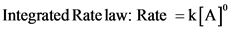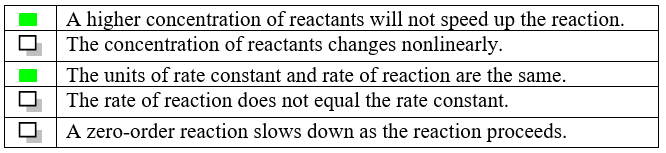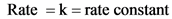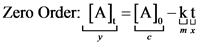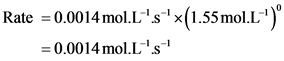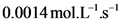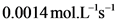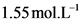# Which of the following are correct for zero order reactionsWhich of the following are correct for zero-order reactions? Check all that apply. A higher concentration of reactants will not speed up the reaction. The concentration of the reactants changes nonlinearly. The units for the rate constant and the rate of reaction are the same. The rate of reaction does not equal the rate constant. A zero-order reaction slows down as the reaction proceeds. In the hydrogenation of ethylene using a nickel catalyst, the initial concentration of ethylene is 1.55mol middot L-1 and its rate constant (k) is 0.0014mol middot L-1 middot s-1. Determine the rate of reaction if it follows a zero-order reaction mechanism. Express your answer to two significant figures and include the appropriate units.

General guidance

Concepts and reason
The given problem is based on the kinetic study of the rate of the reaction. In the first part of the problem correct statement regarding the zero-order reaction needs to be marked and in the second part of the problem, rate of reaction needs to be calculated using given initial rate and rate constant.
Firstly, write the expression for the zero-order reaction then, identify which statements follows the equation and then substitute the values in the rate law expression to find the rate.

Fundamentals

Consider both the differential and integrated equation for the zero-order of reaction as shown below:

Here,

Step-by-step

Step 1 of 2

A)
Consider the rate law expression as follows:

From the above expression, following statement are true:
• Rate of Zero-order reaction does not depend on the concentration of reactant as the rate law expression contains the zero power of the reactant concentration.
• Units of rate and rate constant will be same as both are equal for zero order reaction.

Part A
Part A
Hence, the correct statements are marked as follows:

Rate of Zero-order reaction does not depend on the concentration of reactant as the rate law expression contains the zero power of the reactant concentration. Hence, the concentration of reactant will not affect the rate of reaction.
Therefore, first statement given in the table is true while the last statement is false.
Now, rewrite the rate law expression as shown below:

Therefore, units of rate and rate constant will be same as both are equal for zero order reaction. Hence, third statement is true while fourth statement is false.
Now, consider the differential rate law expression as shown below:

Here, y is the y-axis intercept, x is the x-axis intercept, m is the slope, and c is the constant.
From the above equation, the concentration of reactant changes linearly as it is following the straight-line equation. Hence, second statement is false.

Draw the correct inference from the rate law expression and do get confuse by seeing the concentration term in the rate law expression. Zero power of anything is equivalent to one.

Calculate the rate of the reaction by using the rate law expression.

Step 2 of 2

B)
Use integrated rate law expression to find the rate of reaction as shown below:

Part B
The rate of reaction of the given zero order reaction is .

Consider the rate law expression as follows:

Substitute for k and for , that is, concentration of in above formula as shown below:

Rate of reaction for zero order reaction is equal to the rate constant.

Use the correct formula and place the units correctly.

Part A
Part A
Hence, the correct statements are marked as follows:

Part B
The rate of reaction of the given zero order reaction is .

Part A
Part A
Hence, the correct statements are marked as follows:

Part B
The rate of reaction of the given zero order reaction is .

Differential rate law: [A]. = [A]. – kt Integrated Rate law: Rate = k[A]º
[A] = concentration at timet=t [A] = concentration at timet = 0 k=rate constant t = time
Integrated Rate law: Rate = k[A]º
Rate = k = rate constant
17 -“Tv]=”[v] :10p10 0127
Rate = 0.0014 mol.l-‘s ‘x(1.55 mol.L””)” = 0.0014 mol.l’s
Integrated Rate law: Rate = k[A]º
0.0014 mol.l’s
1.55 mol.L’
Rate = 0.0014 mol.l-‘s ‘x(1.55 mol.L””)” = 0.0014 mol.l’s
A higher concentration of reactants will not speed up the reaction. The concentration of reactants changes nonlinearly. The units of rate constant and rate of reaction are the same. | The rate of reaction does not equal the rate constant. A zero-order reaction slows down as the reaction proceeds. TO D
A higher concentration of reactants will not speed up the reaction. The concentration of reactants changes nonlinearly. The units of rate constant and rate of reaction are the same. | The rate of reaction does not equal the rate constant. A zero-order reaction slows down as the reaction proceeds. TO D
O A higher concentration of reactants will not speed up the reaction. The concentration of reactants changes nonlinearly. The units of rate constant and rate of reaction are the same. The rate of reaction does not equal the rate constant. A zero-order reaction slows down as the reaction proceeds.
A higher concentration of reactants will not speed up the reaction. The concentration of reactants changes nonlinearly. | The units of rate constant and rate of reaction are the same. The rate of reaction does not equal the rate constant. A zero-order reaction slows down as the reaction proceeds.
We were unable to transcribe this image